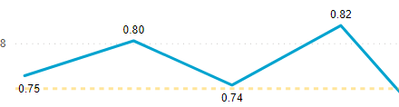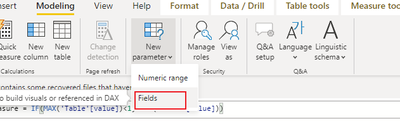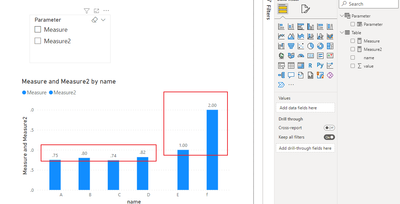cancel
Showing results for
Did you mean:Frequent Visitor

## How can I remove leading zeroes from the data labels?

Hello! I'd like to show this calculated measure in the data labels in the format '.XX' (i.e. '.76' as opposed to '0.76') if <1 and just '1' if =1. Basically removing non-significant zeroes. I tried using RIGHT, then converting back to a Decimal number but got a bad output. Thanks!1 ACCEPTED SOLUTIONHelper I

Hi, @beemunni ;

You could create measure.

``Measure = IF(MAX('Table'[value])<1, MAX('Table'[value]))``
``Measure2 = IF(MAX('Table'[value])>=1 ,MAX('Table'[value]))``

and change the measure format.Then create a feild parameter.The final show:Best Regards,

3 REPLIES 3Helper I

Hi, @beemunni ;

You could create measure.

``Measure = IF(MAX('Table'[value])<1, MAX('Table'[value]))``
``Measure2 = IF(MAX('Table'[value])>=1 ,MAX('Table'[value]))``

and change the measure format.Then create a feild parameter.The final show:Best Regards,Super User

Try this

FORMAT(tablename[fieldname],"#.##")

Thanks for reaching out for help.

I put in a lot of effort to help you, now please quickly help me by giving kudos.

Remeber we are unpaid volunteers and here to coach you with Power BI and DAX skills and techniques, not do the users job for them. So please click the thumbs up and accept as solution button.

If you give someone a fish then you only give them one meal, but if you teach them how to fish then they can feed themselves and teach others for a lifetime.  I prefer to teach members on this forum techniques rather give full solutions and do their job. You can then adapt the technique for your solution, learn some DAX skills for next time and soon become a Power BI Super User like me.

One question per ticket please. If you need to extend your request then please raise a new ticket.

You will get a quicker response and each volunteer solver will get the kudos they deserve. Thank you !Frequent Visitor

That gave me the error, "Error Message:
Query (39, 5) The SampleAxisWithLocalMinMax function requires Measure parameter (at argument number 5) to be a numeric column reference from the specified table." The line graph Can't Display because that function converts to text.